# Equation with abs

Solve this equation with absolute value member:

$|3(3x-1)|=8$

Correct result:

x =  1.2222
y =  -0.5556

#### Solution:

3•(3x-1)=8

3•(3•x-1)=8

9x = 11

x = 11/9 ≈ 1.222222

Our simple equation calculator calculates it.

-3•(3y-1)=8

-3•(3•y-1)=8

9y = -5

y = -5/9 ≈ -0.555556

Our simple equation calculator calculates it.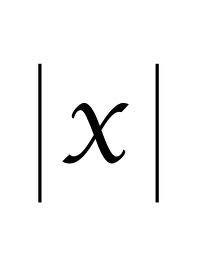We would be pleased if you find an error in the word problem, spelling mistakes, or inaccuracies and send it to us. Thank you!Tips to related online calculators
Do you have a linear equation or system of equations and looking for its solution? Or do you have quadratic equation?

## Next similar math problems:

• ABS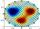What is the value of ? ?
• Eq with reciprocalSolve given equation with reciprocal member: a-6/a+10=4/8
• Equation with abs value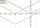How many solutions has the equation ? in the real numbers?
• Nineteenth memberFind the nineteenth member of the arithmetic sequence: a1=33 d=5 find a19
• Fifth memberDetermine the fifth member of the arithmetic progression, if the sum of the second and fifth members equal to 73, and difference d = 7.
• SequenceIn the arithmetic sequence is a1=-1, d=4. Which member is equal to the number 203?
• EQ2Solve quadratic equation: ?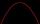Solve pure quadratic equation ?.
• Probably memberLook at the series 2,6,25,96,285, ? What number should come next?
• AlgebrogramSolve algebrogram for sum of three numbers: BEK KEMR SOMR ________ HERCI
• Arithmetic progression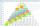In some AP applies: 5a2 + 7a5 = 90 s3 = 12 Find the first member a =? and difference d = ?
• Solve equationsolve equation: ?
• Vectors abs sum diff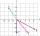The vectors a = (4,2), b = (- 2,1) are given. Calculate: a) |a+b|, b) |a|+|b|, c) |a-b|, d) |a|-|b|.
• Equation - inverseSolve for x: 7: x = 14: 1000Solve quadratic equation: 2x2+28x-550=0Determine the third member of the AP if a4=93, d=7.5.Solve for x: (4^x):0,5=2/64.